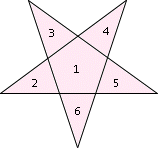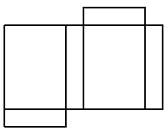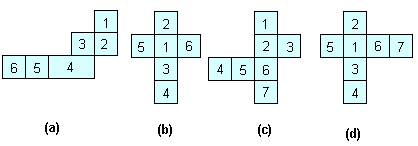## Definition Of Nine Point Circle

Net is a two-dimensional pattern of a three-dimensional figure that can be folded to form the figure.

In other words, net is a flattened three-dimensional figure which can be turned into the solid by folding it.

### Examples of Net

The following is the net of a pentagonal pyramid.The following is the net of a rectangular prism.### Solved Example on Net

#### Ques: Which of the nets can be folded to form a cube?##### Choices:

A. Net (c)
B. Net (b) and Net (c)
C. Net (b), Net (c), and Net (d)
D. Net (b)